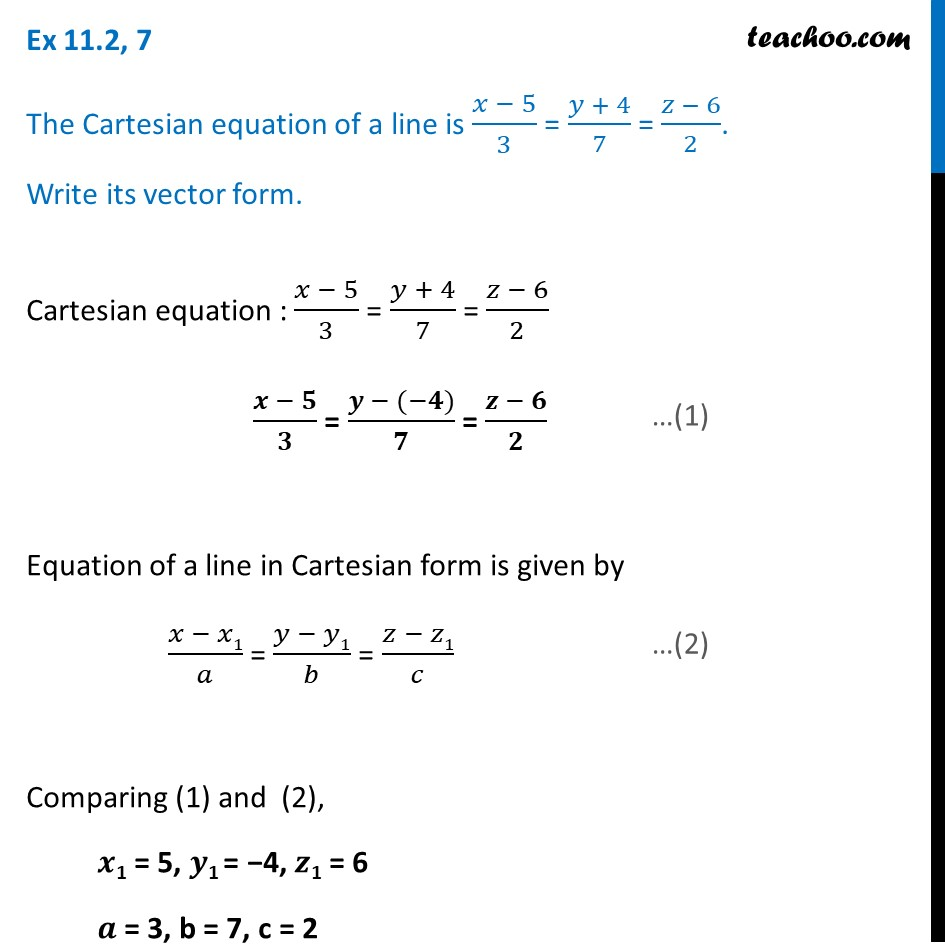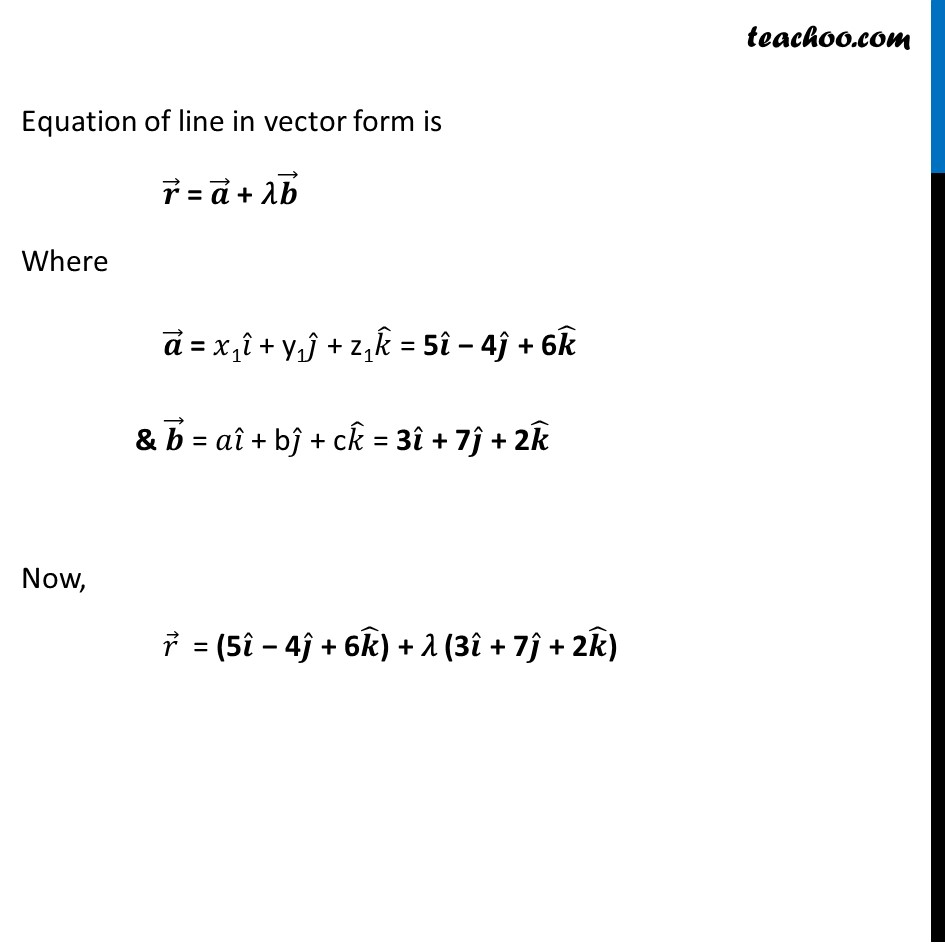Ex 11.2

Chapter 11 Class 12 Three Dimensional Geometry
Serial order wiseLearn in your speed, with individual attention - Teachoo Maths 1-on-1 Class

### Transcript

Ex 11.2, 7 The Cartesian equation of a line is (𝑥 − 5)/3 = (𝑦 + 4)/7 = (𝑧 − 6)/2. Write its vector form.Cartesian equation : (𝑥 − 5)/3 = (𝑦 + 4)/7 = (𝑧 − 6)/2 (𝒙 − 𝟓)/𝟑 = (𝒚 − (−𝟒))/𝟕 = (𝒛 − 𝟔)/𝟐 Equation of a line in Cartesian form is given by (𝑥 − 𝑥1)/𝑎 = (𝑦 − 𝑦1)/𝑏 = (𝑧 − 𝑧1)/𝑐 Comparing (1) and (2), 𝒙1 = 5, 𝒚1 = −4, 𝒛1 = 6 𝒂 = 3, b = 7, c = 2 Equation of line in vector form is 𝒓 ⃗ = 𝒂 ⃗ + 𝜆𝒃 ⃗ Where 𝒂 ⃗ = 𝑥1𝑖 ̂ + y1𝑗 ̂ + z1𝑘 ̂ = 5𝒊 ̂ − 4𝒋 ̂ + 6𝒌 ̂ & 𝒃 ⃗ = 𝑎𝑖 ̂ + b𝑗 ̂ + c𝑘 ̂ = 3𝒊 ̂ + 7𝒋 ̂ + 2𝒌 ̂ Now, 𝑟 ⃗ = (5𝒊 ̂ − 4𝒋 ̂ + 6𝒌 ̂) + 𝜆 (3𝒊 ̂ + 7𝒋 ̂ + 2𝒌 ̂)